The RELIABILITY Procedure
 PROBPLOT Statement
<label:>PROBPLOT variable<*censor-variable(values)> <=group-variables> </options> ;
<label:>PROBPLOT (variable1 variable2) <=group-variables> </options> ;

You use the PROBPLOT statement to create a probability plot from complete, left-censored, right-censored, or interval-censored data.

You can specify the keyword PPLOT as an alias for PROBPLOT. You can specify any number of PROBPLOT statements after a PROC RELIABILITY statement. Each PROBPLOT statement creates a probability plot and an associated analysis. The probability distribution used in creating the probability plot and performing the analysis is determined by the DISTRIBUTION statement. You can specify an optional label to distinguish between multiple PROBPLOT statements in the output.

See the section Analysis of Right-Censored Data from a Single Population and the section Weibull Analysis Comparing Groups of Data for examples that create probability plots with the PROBPLOT statement.

To create a probability plot, you must specify one variable. If your data are right censored, you must specify a censor-variable and, in parentheses, the values of the censor-variable that correspond to censored data values.

You can optionally specify one or two group-variables (also referred to as classification variables). The PROBPLOT statement displays a probability plot for each level of the group-variables. The observations in a given level are called a cell.

The elements of the PROBPLOT statement are described as follows.

variable

represents the data for which a probability plot is to be produced. The variable must be a numeric variable in the input data set.

censor-variable(values)

indicates which observations in the input data set are right censored. You specify the values of censor-variable that represent censored observations by placing those values in parentheses after the variable name. If your data are not right censored, then you can omit the specification of censor-variable; otherwise, censor-variable must be a numeric variable in the input data set.

(variable1 variable2)

is another method of specifying the data for which a probability plot is to be produced. You can use this syntax in a situation where uncensored, interval-censored, left-censored, and right-censored values occur in the same set of data. Table 12.26 shows how you use this syntax to specify different types of censoring by using combinations of missing and nonmissing values. See the section Lognormal Analysis with Arbitrary Censoring for an example that uses this syntax to create a probability plot.

group-variables

are one or two group variables. If no group variables are specified, a single probability plot is produced. The group-variables can be numeric or character variables in the input data set.

Note that the parentheses surrounding the group-variables are needed only if two group variables are specified.

options

control the features of the probability plot. All options are specified after the slash (/) in the PROBPLOT statement. See the section Summary of Options, which follows, for a list of all options by function.

Summary of Options

Table 12.29 Analysis Options

Option

Option Description

CONFIDENCE=number

specifies the confidence coefficient for all confidence intervals. The number must be between 0 and 1. The default value is 0.95

CONVERGE=number

specifies the convergence criterion for maximum likelihood fit. See the section Maximum Likelihood Estimation for details.

CONVH=number

specifies the convergence criterion for the relative Hessian convergence criterion See the section Maximum Likelihood Estimation for details.

CORRB

requests parameter correlation matrix

COVB

requests parameter covariance matrix

FITTYPE | FIT=

specifies method of estimating distribution parameters

LSYX

-least squares fit to the probability plot. The probability axis is the dependent variable.

| LSXY

-least squares fit to the probability plot. The lifetime axis is the dependent variable.

| MLE

-maximum likelihood (default)

| MODEL

-use the fit from the preceding MODEL statement

| NONE

-no fit is computed

| WEIBAYES

-Weibayes method

<(CONFIDENCE|CONF=number)>

number is the confidence coefficient for the Weibayes fit and is between 0 and 1. The default value is 0.95.

ITPRINT

requests iteration history for maximum likelihood fit

ITPRINTEM

requests iteration history for the Turnbull algorithm

LRCL

requests likelihood ratio confidence intervals for distribution parameters

LRCLPER

requests likelihood ratio confidence intervals for distribution percentiles

LOCATION=number <LINIT>

specifies fixed or initial value of location parameter

MAXIT=number

specifies maximum number of iterations allowed for maximum likelihood fit

MAXITEM=number1 <,number2>

number1 specifies maximum number of iterations allowed for Turnbull algorithm. Iteration history will be printed in increments of number2 if requested with ITPRINTEM. See the section Interval-Censored Data for details.

NOPCTILES

suppresses computation of percentiles for standard list of percentage points

NOPOLISH

suppress setting small interval probabilities to zero in the Turnbull algorithm. See the section Interval-Censored Data for details.

NPINTERVALS=

specifies type of nonparametric confidence interval displayed in a probability plot

POINTWISE | POINT

-pointwise confidence intervals for the CDF

| SIMULTANEOUS

| SIMUL<number1, number2>

-simultaneous confidence intervals for the CDF. See the section Simultaneous Confidence Intervals for details.

PCTLIST=number-list

specifies list of percentages for which to compute percentile estimates. The number-list must be a list of numbers separated by blanks or commas. Each number in the list must be between 0 and 100.

PINTERVALS=

type of parametric pointwise confidence interval displayed in a probability plot. The default type is PROBABILITY, pointwise confidence intervals on cumulative failure probability.

LIKELIHOOD | LRCI

-likelihood ratio confidence intervals

| PERCENTILES | PER

-pointwise parametric confidence intervals for the percentiles of the fitted CDF

| PROBABILITY | CDF

-pointwise parametric confidence intervals for the cumulative failure probabilities. See the section Reliability Function for details.

PPOS=

specifies plotting position type. See the section Probability Plotting for details.

EXPRANK

-expected ranks

| MEDRANK

-median ranks

| MEDRANK1

-median ranks (exact formula)

| KM

-Kaplan-Meier

| MKM

-modified Kaplan-Meier (default)

|(NA | NELSONAALEN)

- Nelson-Aalen

PPOUT

request table of cumulative probabilities

PRINTPROBS

print intervals and associated probabilities for the Turnbull algorithm

PROBLIST=number-list

specifies list of initial values for Turnbull algorithm. See the section Interval-Censored Data for details.

PSTABLE=number

specifies stable parameterization. The number must be between zero and one. See the section Stable Parameters for further information.

SCALE=number <SCINIT>

specifies fixed or initial value of scale parameter

SHAPE=number <SHINIT>

specifies fixed or initial value of shape parameter

SINGULAR=number

specifies singularity criterion for matrix inversion

SURVTIME=number-list

requests that the survival function, cumulative distribution function, and confidence limits be computed for values in number-list. See the section Reliability Function for details.

THRESHOLD=number

specifies a fixed threshold parameter. See Table 12.49 for the distributions with a threshold parameter.

TOLLIKE=number

specifies criterion for convergence in the Turnbull algorithm. Default is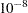. See the section Interval-Censored Data for details.

TOLPROB=number

specifies criterion for setting interval probability to zero in the Turnbull algorithm. Default is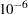. See the section Interval-Censored Data for details.

Table 12.30 lists plot layout options available when you use traditional graphics.

Table 12.30 Probability Plot Layout Options for Traditional Graphics

Option

Option Description

CENBIN

plots censored data as frequency counts rather than as individual points

CENSYMBOL=

symbol | (symbol list)

specifies symbols for censored values. The symbol is one of the symbol names (plus, star, square, diamond, triangle, hash, paw, point, dot, circle) or a letter (A–Z). For overlaid plots for groups of data, you can specify different symbols for the groups with a list of symbols or letters, separated by blanks, enclosed in parentheses. If no CENSYMBOL option is specified, the symbol used for censored values is the same as for failures.

HOFFSET=value

specifies offset for horizontal axis

INBORDER

requests a border around probability plots

INTERTILE=value

specifies distance between tiles

LFIT=linetype | (linetype list))

line styles for fit lines and confidence curves in a probability plot. The linetype list is a list of numbers from 1 to 46 representing different linetypes, and can be separated by blanks or commas or can be a list in the form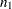to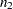<by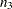>.

MISSING1

requests that missing values of first GROUP= variable be treated as a level of the variable

MISSING2

requests that missing values of second GROUP= variable be treated as a level of the variable

NCOLS=specifies number of columns plotted on a page

NOCENPLOT

suppresses plotting of censored data points

NOCONF

suppresses plotting of percentile confidence curves

NOFIT

suppresses plotting of fit line and percentile confidence curves

NOFRAME

suppresses frame around plotting area

NOINSET

suppresses inset

NOPPLEGEND

suppresses legend for overlaid probability plots

NOPPOS

suppresses plotting of symbols for failures in a probability plot

NROWS=specifies number of rows plotted on a page

ORDER1=DATA | FORMATTED |
FREQ | INTERNAL

specifies display order for values of the first GROUP= variable

ORDER2=DATA | FORMATTED |
FREQ | INTERNAL

specifies display order for values of the second GROUP= variable

OVERLAY

requests overlaid plots for group variables

PCONFPLT

plots confidence intervals on probabilities for readout data

PPLEGEND = legend-statement-name | NONE

identifies LEGENDn statement to specify legend for overlaid probability plots

PPOSSYMBOL=symbol | (symbol list)

symbols representing failures on a probability plot

ROTATE

requests probability plots with probability scale on horizontal axis

SHOWMULTIPLES

display the count for multiple overlaying symbols

TURNVLABELS

vertically strings out characters in labels for vertical axis

VOFFSET=value

length of offset at upper end of vertical axis

WFIT=linetype

line width for fit line and confidence curves

Table 12.31 lists plot layout options available when you use ODS graphics.

Table 12.31 Probability Plot Layout Options for ODS Graphics

Option

Option Description

MISSING1

requests that missing values of first GROUP= variable be treated as a level of the variable

MISSING2

requests that missing values of second GROUP= variable be treated as a level of the variable

NCOLS=specifies number of columns plotted on a page

NOCENPLOT

suppresses plotting of censored data points

NOCONF

suppresses plotting of percentile confidence curves

NOFIT

suppresses plotting of fit line and percentile confidence curves

NOINSET

suppresses inset

NOPPLEGEND

suppresses legend for overlaid probability plots

NOPPOS

suppresses plotting of symbols for failures in a probability plot

NROWS=specifies number of rows plotted on a page

ORDER1=DATA | FORMATTED |
FREQ | INTERNAL

specifies display order for values of the first GROUP= variable

ORDER2=DATA | FORMATTED |
FREQ | INTERNAL

specifies display order for values of the second GROUP= variable

OVERLAY

requests overlaid plots for group variables

PCONFPLT

plots confidence intervals on probabilities for readout data

ROTATE

requests probability plots with probability scale on horizontal axis

Table 12.32 lists reference line options available when you use traditional graphics.

Table 12.32 Reference Line Options for Traditional Graphics

Option

Option Description

HREF <(INTERSECT)>=value-list

requests reference lines perpendicular to horizontal axis. If (INTERSECT) is specified, a second reference line perpendicular to the vertical axis is drawn that intersects the fit line at the same point as the horizontal axis reference line. If a horizontal axis reference line label is specified, the intersecting vertical axis reference line is labeled with the vertical axis value.

HREFLABELS=(label1’ ...’labeln)

specifies labels for HREF= lines

HREFLABPOS=specifies vertical position of labels for HREF= lines. The valid values for n and the corresponding label placements are shown in the following table:

n

label placement

1

top

2

staggered from top

3

bottom

4

staggered from bottom

5

alternating from top

6

alternating from bottom

LHREF=linetype

specifies line style for HREF= lines

LVREF=linetype

specifies line style for VREF= lines

VREF <(INTERSECT)>=value-list

specifies reference lines perpendicular to vertical axis. If (INTERSECT) is specified, a second reference line perpendicular to the horizontal axis is drawn that intersects the fit line at the same point as the vertical axis reference line. If a vertical axis reference line label is specified, the intersecting horizontal axis reference line is labeled with the horizontal axis value.

VREFLABELS=(label1’ ...’labeln)

specifies labels for VREF= lines

VREFLABPOS=specifies horizontal position of labels for VREF= lines. The valid values for n and the corresponding label placements are shown in the following table:

n

label placement

1

left

2

right

Table 12.33 lists reference line options available when you use ODS graphics.

Table 12.33 Reference Line Options for ODS Graphics

Option

Option Description

HREF |

LREF <(INTERSECT)>=value-list

requests reference lines perpendicular to horizontal axis. If (INTERSECT) is specified, a second reference line perpendicular to the vertical axis is drawn that intersects the fit line at the same point as the horizontal axis reference line. If a horizontal axis reference line label is specified, the intersecting vertical axis reference line is labeled with the vertical axis value.

HREFLABELS |

LREFLABELS =(label1’ ...’labeln)

specifies labels for HREF= lines

VREF |

PREF <(INTERSECT)>=value-list

specifies reference lines perpendicular to vertical axis. If (INTERSECT) is specified, a second reference line perpendicular to the horizontal axis is drawn that intersects the fit line at the same point as the vertical axis reference line. If a vertical axis reference line label is specified, the intersecting horizontal axis reference line is labeled with the horizontal axis value.

VREFLABELS |

PREFLABELS=(label1’ ...’labeln)

specifies labels for VREF= lines

The following options control the appearance of the text when you use traditional graphics. These options are not available if ODS Graphics is enabled.

Table 12.34 Text Enhancement Options

Option

Option Description

FONT=font

specifies a software font for text

HEIGHT=value

specifies height of text used outside framed areas

INFONT=font

specifies a software font for text inside framed areas

INHEIGHT=value

specifies height of text inside framed areas

Table 12.35 lists options to control the appearance of the axes when you use traditional graphics.

Table 12.35 Axis Options for Traditional Graphics

Option

Option Description

LAXIS=value1 to value2<by value3>

specifies tick mark values for the lifetime axis. value1, value2, and value3 must be numeric, and value1 must be less than value2. The lower tick mark is value1. Tick marks are drawn at increments of value3. The last tick mark is the greatest value that does not exceed value2. If value3 is omitted, a value of 1 is used. This method of specification of tick marks is not valid for logarithmic axes. Examples of LAXIS= lists are

```laxis = -1 to 10
laxis = 0 to 200 by 10
```

LGRID=number

specifies a line style for all grid lines. The number is between 1 and 46 and specifies a linestyle for grids.

LIFELOWER | LLOWER=number

specifies the lower limit on the lifetime axis scale. The LLOWER option specifies number as the lower lifetime axis tick mark. The tick interval and the upper lifetime axis limit are determined automatically. This option has no effect if the LAXIS option is used.

LIFEUPPER | LUPPER=number

specifies the upper limit on the lifetime axis scale. The LUPPER option specifies number as the upper lifetime axis tick mark. The tick interval and the lower lifetime axis limit are determined automatically. This option has no effect if the LAXIS option is used.

MPGRID

adds a minor grid for the probability axis

MINORLOGGRID

adds a minor grid for log axes

NOGRID

suppresses grid lines

NOLLABEL

suppresses label for life, or analysis variable, axis

NOLTICK

suppresses tick marks and tick mark labels for lifetime or analysis variable axis

NOPLABEL

suppresses label for probability axis

NOPTICK

suppresses tick marks and tick mark labels for the probability axis

NTICK=number

specifies the number of tick intervals for the lifetime axis. This option has no effect if the LAXIS option is used.

PCTLOWER | PLOWER=number

specifies the lower limit on probability axis scale

PCTUPPER | PUPPER=number

specifies the upper limit on probability axis scale

PAXISLABEL=‘string

specifies a label for the probability axis

WAXIS=specifies the line thickness for axes and frame

Table 12.36 lists options to control the appearance of the axes when you use ODS graphics.

Table 12.36 Axis Options for ODS Graphics

Option

Option Description

LIFELOWER | LLOWER=number

specifies the lower limit on the lifetime axis scale. The LLOWER option specifies number as the lower lifetime axis tick mark. The tick interval and the upper lifetime axis limit are determined automatically. This option has no effect if the LAXIS option is used.

LIFEUPPER | LUPPER=number

specifies the upper limit on the lifetime axis scale. The LUPPER option specifies number as the upper lifetime axis tick mark. The tick interval and the lower lifetime axis limit are determined automatically. This option has no effect if the LAXIS option is used.

MINORLOGGRID

adds a minor grid for log axes

NOGRID

suppresses grid lines

PCTLOWER | PLOWER=number

specifies the lower limit on probability axis scale

PCTUPPER | PUPPER=number

specifies the upper limit on probability axis scale

PAXISLABEL=‘string

specifies a label for the probability axis

The following options control colors and patterns used in the graph when you use traditional graphics. These options are not available if ODS Graphics is enabled.

Table 12.37 Color and Pattern Options

Option

Option Description

CAXIS=color

color for axis

CCENSOR=color

color for filling censor plot area

CENCOLOR=color

color for censor symbol

CFIT=color | (color list)

color for fit lines and confidence curves in a probability plot

CFRAME=color

color for frame

CFRAMESIDE=color

color for filling frame for row labels

CFRAMETOP=color

color for filling frame for column labels

CGRID=color

color for grid lines

CHREF=color

color for HREF= lines

CTEXT=color

color for text

CVREF=color

color for VREF= lines

PPOSCOLOR=color | (color list)

colors of symbols representing failures on a probability plot

The following options control the use of a graphics catalog to store graphs if you use traditional graphics. These options are not available if ODS Graphics is enabled.

Table 12.38 Graphics Catalog Options

Option

Option Description

DESCRIPTION=‘string

description for graphics catalog member

NAME=‘string

name for plot in graphics catalogPrevious Page | Next Page | Top of Page